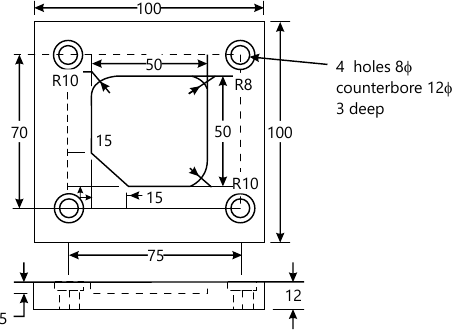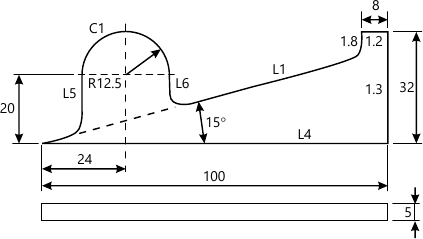MU Mechanical Engineering (Semester 7)
May 2014
Total marks: --
Total time: --
INSTRUCTIONS
(1) Assume appropriate data and state your reasons
(2) Marks are given to the right of every question
(3) Draw neat diagrams wherever necessary

1 (a) Explain CAD tools required to support the different design phases
6 M
7 M
1 (c) A cubic Bezier curve is defined by the control points as (20, 20), (60, 80), (120,100) and (150, 30). Find the equation of the curve and Its mid point.
7 M

2 (a) Write a part program using G and M codes to machine the contour as shown in Figure 1 and drill holes. The component is 18 mm thick.Assume
(i) Suitable tool
(ii) Suitable speed and feed for machining.
10 M
2 (b) A rectangle ABCD has vertices A(1,1), B(2,1), C(2,3), DU ,3) . It has to to be rotated by 30° CCW about point P (3, 2). Determine: (i) the composite transformation matrix (ii) the new coordinates of rectangle.
10 M

3 (a) Explain any one hidden line removal algorithm and explain how the algorithm determines which entities are hidden
10 M
3 (b) Explain contact and non-contact inspection methods in Computer Aided Quality Control (CAQC)
10 M

4 (a) Explain any two rapid prototyping processes
10 M
4 (b) Write a complete APT program (geometric and motion commands) to machine the outline of the geometry as shown in Figure 2. Assume suitable data.10 M

5 (a) Write a program in object oriented language for 2D transformation which include functions for the following operations: (1) rotation @ x-axis (ii) scaling
10 M
5 (b) Explain the CIM wheel with neat sketch along with the features and functioning of CIM systems
10 M

6 (a) Explain pan classification and coding in Group Technology in detail
7 M
6 (b) Explain similarity coefficient matrix
3 M
6 (c) List benefits using Artificial Intelligence in CAPP system
5 M
6 (d) Write short note on design for assembly
5 M

Write short note on (any two)
7 (i) Automated material handling system.
10 M
7 (ii) Wire frame modelling
10 M
7 (iii) Light and shade ray tracing
10 M
7 (iv) 3D rotation transformation
10 M

More question papers from CAD / CAM / CIM